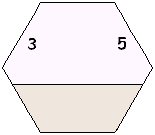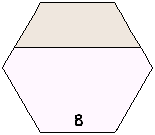* How to use the cards * Print the cards * Games and activities
* How to use the fact family cards
Each card has three numbers on it. The number at the bottom is the sum of the two numbers above it. These three numbers are a fact family. This card shows four related facts: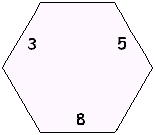3 + 5 = 8
5 + 3 = 8
8 - 3 = 5
8 - 5 = 3
You can cut and glue a "fact family house" to hide one number at a time.
Place a stack of fact family cards in the house, say the missing number on the card (see the examples below), then pull the card off the stack and go on to the next card. At any time, you can move the position of the house so that it is hiding a different side of the cards.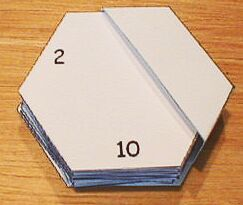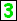+ 5 = 8What plus 5 is 8?8 - 5 =What is 8 - 5?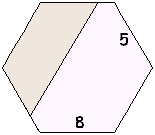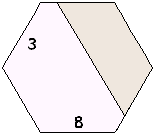3 +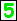= 83 plus what is 8?8 - 3 =What is 8 - 3?3 + 5 =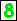3 plus 5 is what?- 3 = 5 What - 3 = 5?- 5 = 3 What - 5 = 3?